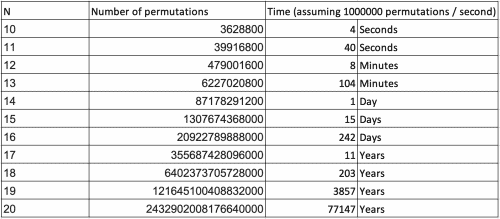### Get started with Spring and Spring Boot, through the Learn Spring course:

>> CHECK OUT THE COURSE

## 1. Introduction

In this article, we’ll look at how to create permutations of an array.

First, we’ll define what a permutation is. Second, we’ll look at some constraints. And third, we’ll look at three ways to calculate them: recursively, iteratively, and randomly.

We’ll focus on the implementation in Java and, therefore, won’t go into a lot of mathematical detail.

## 2. What Is a Permutation?

A permutation of a set is a rearrangement of its elements. A set which consists of n elements has n! permutations. Here n! is the factorial, which is the product of all positive integers smaller or equal to n.

### 2.1. Example

The array of integers [3,4,7] has three elements and six permutations:

n! = 3! = 1 x 2 x 3 = 6

Permutations: [3,4,7]; [3,7,4]; [4,7,3]; [4,3,7]; [7,3,4]; [7,4,3]

### 2.2. Constraints

The number of permutation increases fast with n. While it takes only a few seconds to generate all permutations of ten elements, it will take two weeks to generate all permutations of 15 elements:## 3. Algorithms

### 3.1. Recursive Algorithm

The first algorithm we look at is Heap’s algorithm. It’s a recursive algorithm which produces all permutations by swapping one element per iteration.

The input array will be modified. If we don’t want that, we need to create a copy of the array before calling the method:

``````public static <T> void printAllRecursive(
int n, T[] elements, char delimiter) {

if(n == 1) {
printArray(elements, delimiter);
} else {
for(int i = 0; i < n-1; i++) {
printAllRecursive(n - 1, elements, delimiter);
if(n % 2 == 0) {
swap(elements, i, n-1);
} else {
swap(elements, 0, n-1);
}
}
printAllRecursive(n - 1, elements, delimiter);
}
}
``````

The method uses two helper methods:

``````private static void swap(T[] elements, int a, int b) {
T tmp = elements[a];
elements[a] = elements[b];
elements[b] = tmp;
}``````
``````private static void printArray(T[] elements, char delimiter) {
String delimiterSpace = delimiter + " ";
for(int i = 0; i < elements.length; i++) {
System.out.print(elements[i] + delimiterSpace);
}
System.out.print('\n');
}
``````

Here, we write the result to System.out, however, we can easily store the result in an array or in a list instead.

### 3.2. Iterative Algorithm

Heap’s algorithm can also be implemented using iterations:

``````int[] indexes = new int[n];
int[] indexes = new int[n];
for (int i = 0; i < n; i++) {
indexes[i] = 0;
}

printArray(elements, delimiter);

int i = 0;
while (i < n) {
if (indexes[i] < i) {
swap(elements, i % 2 == 0 ?  0: indexes[i], i);
printArray(elements, delimiter);
indexes[i]++;
i = 0;
}
else {
indexes[i] = 0;
i++;
}
}
``````

### 3.3. Permutations in Lexicographical Order

If the elements are comparable, we can generate permutations sorted by the natural order of the elements:

``````public static <T extends Comparable<T>> void printAllOrdered(
T[] elements, char delimiter) {

Arrays.sort(elements);
boolean hasNext = true;

while(hasNext) {
printArray(elements, delimiter);
int k = 0, l = 0;
hasNext = false;
for (int i = elements.length - 1; i > 0; i--) {
if (elements[i].compareTo(elements[i - 1]) > 0) {
k = i - 1;
hasNext = true;
break;
}
}

for (int i = elements.length - 1; i > k; i--) {
if (elements[i].compareTo(elements[k]) > 0) {
l = i;
break;
}
}

swap(elements, k, l);
Collections.reverse(Arrays.asList(elements).subList(k + 1, elements.length));
}
}
``````

This algorithm has a reverse operation in every iteration; therefore, it is less efficient on arrays than Heap’s algorithm.

### 3.4. Randomized Algorithm

If n is big, we can generate a random permutation by shuffling the array:

``Collections.shuffle(Arrays.asList(elements));``

We can do this several times to generate a sample of permutations.

We might create the same permutations more than once, however, for big values of n, the chances to generate the same permutation twice are low.

## 4. Conclusion

There are many ways to generate all permutations of an array. In this article, we saw the recursive and iterative Heap’s algorithm and how to generate a sorted list of permutations.

It’s not feasible to generate all permutations for large arrays, therefore, we can generate random permutations instead.

The implementation of all code snippets in this article can be found in our Github repository.

### Get started with Spring and Spring Boot, through the Learn Spring course:

>> CHECK OUT THE COURSE